/
/
/
141Determine the x and y components of F1 and F2. 142Determine
Not my Question
Flag Content

# Question : 141Determine the x and y components of F1 and F2. 142Determine : 2016496

141Determine the x and y components of F1 and F2.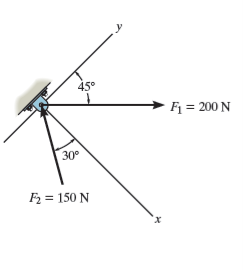142Determine the magnitude of the resultant force and itsdirection, measured counterclockwise from the positivex axis.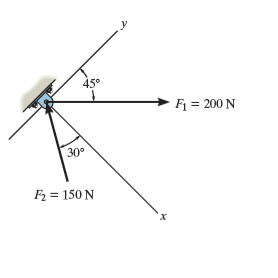143Resolve the 250-N force into components acting along the u and v axes and determine the magnitudes of

these components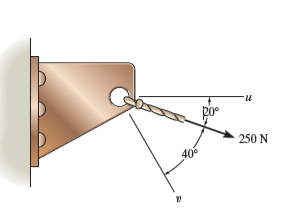144Express F1 and F2 as Cartesian vectors.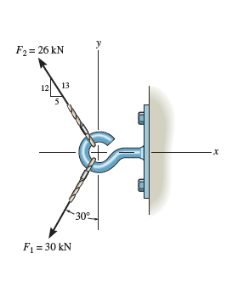145Determine the magnitude of the resultant force and itsdirection measured counterclockwise from the positive ,x axis.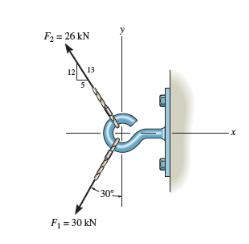146Cable AB exerts a force of 80 N on the end of the3-m-long boom OA. Determine the magnitude of the

projection of this force along the boom.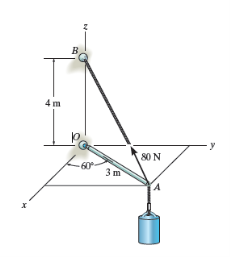147Determine the magnitude and direction of the resultant FR = F1 + F2 + F3 of the three forces by first finding theresultant FR = F1 + F3 and then forming . FR = F + F2Specify its direction measured counterclockwise from the positive x axis.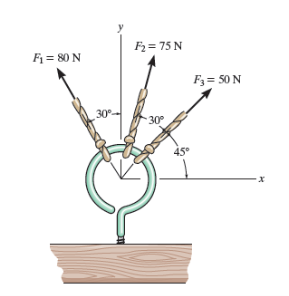148If ? = 60' and F = kN determine the magnitude of theresultant force and its direction measured clockwise fromthe positive x axis.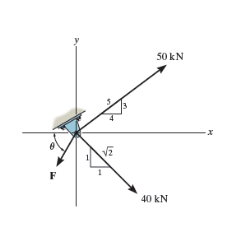149Determine the design angle u (0°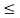u90°) forstrut AB so that the 400-N horizontal force has a

component of 500 N directed from A towards C.What is thecomponent of force acting along member ? = AB? Take

f = 40°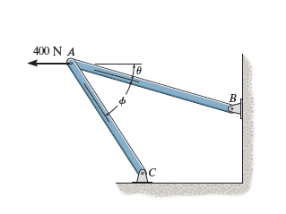## Solution 5 (1 Ratings )

Solved
Electrical Engineering 1 Week Ago 118 Views